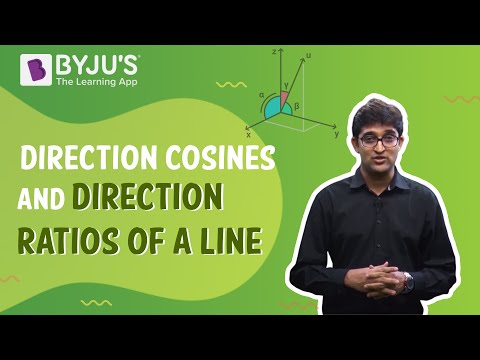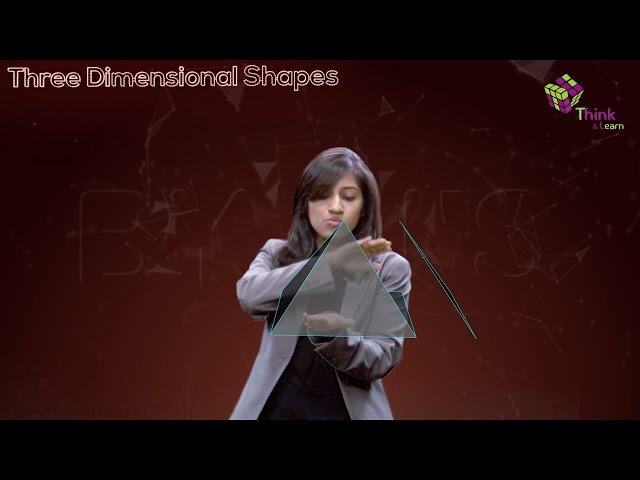# Three Dimensional Geometry Class 12 Notes Chapter 11

## Direction Cosine of Line

This is referred to the cosine of the angles subtended by a line on the positive direction of the coordinate axis. If we are given a line whose direction cosines are p,q,r, then

$$\begin{array}{l}p^{2}+q^{2}+r^{2}=1\end{array}$$
and suppose we have line joining two points such as R(
$$\begin{array}{l}x_{1},y_{1},z_{1}\end{array}$$
) and S(
$$\begin{array}{l}x_{2},y_{2},z_{2}\end{array}$$
) are represented by
$$\begin{array}{l}x_{2}-x_{1}/RS,y_{2}-y_{1}/RS,z_{2}-z_{1}/RS\end{array}$$
and

RS=

$$\begin{array}{l}\sqrt{(x_{2}-x_{1})^{2}+(y_{2}-y_{1})^{2}+(z_{2}-z_{1})^{2}}\end{array}$$

If a line has p,q,r are the direction cosines and a,b,c are the direction ratios then,

p=

$$\begin{array}{l}a/\sqrt{a^{2}+b^{2}+c^{2}}\end{array}$$
,

q=

$$\begin{array}{l}b/\sqrt{a^{2}+b^{2}+c^{2}}\end{array}$$
,

r=

$$\begin{array}{l}c/\sqrt{a^{2}+b^{2}+c^{2}}\end{array}$$

#### For More Information On Direction Cosine Of A Line, Watch The Below Video.### What are Skew Lines?

These are referred to the lines in space which neither intersecting nor parallel. They exist in a different plane. The angle between two lines which intersect each other can be found from any point which is parallel to each of the skew lines.

If

$$\begin{array}{l}p_{1},q_{1},r_{1}\end{array}$$
and
$$\begin{array}{l}p_{2},q_{2},r_{2}\end{array}$$
are the given direction cosines and angle between two distinct lines is
$$\begin{array}{l}\theta\end{array}$$
then,
$$\begin{array}{l}cos\theta\end{array}$$
=
$$\begin{array}{l}\left | p_{1}p_{2}+q_{1}q_{2}+r_{1}r_{2} \right |\end{array}$$

And if two lines have direction ratios as

$$\begin{array}{l}l_{1},m_{1},n_{1}\end{array}$$
and
$$\begin{array}{l}l_{2},m_{2},n_{2}\end{array}$$
and angle
$$\begin{array}{l}\theta\end{array}$$
is given then,
$$\begin{array}{l}\left | l_{1}l_{2}+m_{1}m_{2}+n_{1}n_{2} \right/\sqrt{l_{1}^{2}+m_{1}^{2}+n_{1}^{2} \sqrt{l_{2}^{2}+m_{2}^{2}+n_{2}^{2}}}\end{array}$$

### The equation of the vector of a line

The equation of vector of the line passing through a point having position vector as

$$\begin{array}{l}\underset{a}{\rightarrow}\end{array}$$
and is parallel to a given vector
$$\begin{array}{l}\underset{b}{\rightarrow}\end{array}$$
is
$$\begin{array}{l}\underset{r}{\rightarrow}\end{array}$$
=
$$\begin{array}{l}\underset{a}{\rightarrow}+\lambda \underset{b}{\rightarrow}\end{array}$$
Equation of a plane which is at a distance of d from the origin and the direction
cosines of the normal to the plane as l, m, n is lx + my + nz = d and Shortest distance between two skew lines is the line segment perpendicular to both the lines.

### Cartesian Equation of a Line passing through two points

If there are two points with dimensions

$$\begin{array}{l}(x_{1},y_{1},z_{1}) and (x_{2},y_{2},z_{2})\end{array}$$
then
$$\begin{array}{l}x-x_{1}/x_{2}-x_{1}=y-y_{1}/y_{2}-y_{1}=z-z_{1}/z_{2}-z_{1}\end{array}$$
 Also Access NCERT Solutions for Class 12 Maths Chapter 11 NCERT Exemplar for Class 12 Maths Chapter 11

### Important Questions

1. Distance between the two planes with dimensions 2x + 3y + 4z = 4 and 4x + 6y + 8z = 12 is

(A) 2 units

(B) 4 units

(C) 8 units

(D)

$$\begin{array}{l}2/\sqrt{29}\end{array}$$
units

2. If P be the origin and the coordinates of P be (2, 3, – 4), then find the equation of the plane passing through Q and perpendicular to PQ.

3. Find the coordinates of the point where the line through (4, – 5, – 6) and (3, – 4, 2) crosses the plane 3x + y + z = 8.

4. Find the coordinates of the point where the line through (6, 2, 7) and (4, 5,2) crosses the YZ-plane

5. Find the angle between the lines whose direction ratios are a, b, c and b – c, c – a, a – b.

 Equation Of A Line Equation Of  A Line In Three Dimensions

## Frequently asked Questions on CBSE Class 12 Maths Notes Chapter 11: 3D Geometry

Q1

### What is a ‘Coordinate’ point?

The coordinates of a point are a pair of numbers that define its exact location on a two-dimensional plane.

Q2

### What is ‘Dimensional plane’?

In mathematics, a plane is a flat, two-dimensional surface that extends indefinitely.

Q3

### What is ‘3D geometry’?

3D geometry involves the mathematics of shapes in 3D space and involving 3 coordinates which are x-coordinate, y-coordinate and z-coordinate.